# Magnetic Field Questions and Answers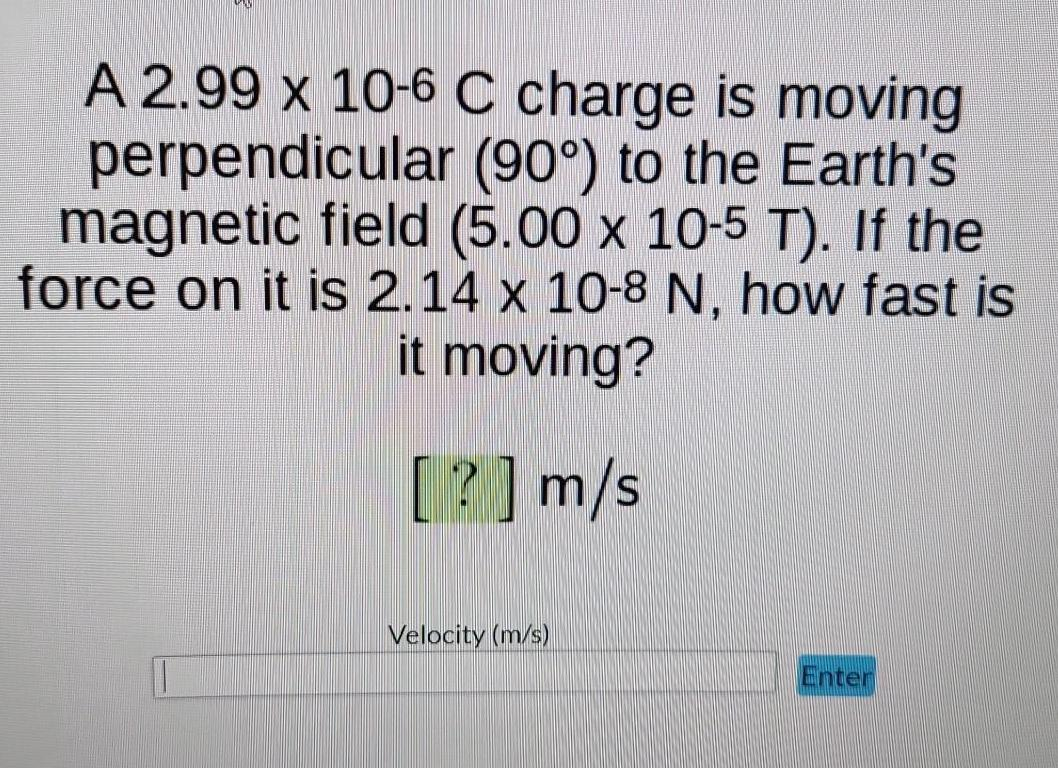Physics
Magnetic Field
A 2.99 x 10-6 C charge is moving perpendicular (90°) to the Earth's magnetic field (5.00 x 10-5 T). If the force on it is 2.14 x 10-8 N, how fast is it moving? [?] m/s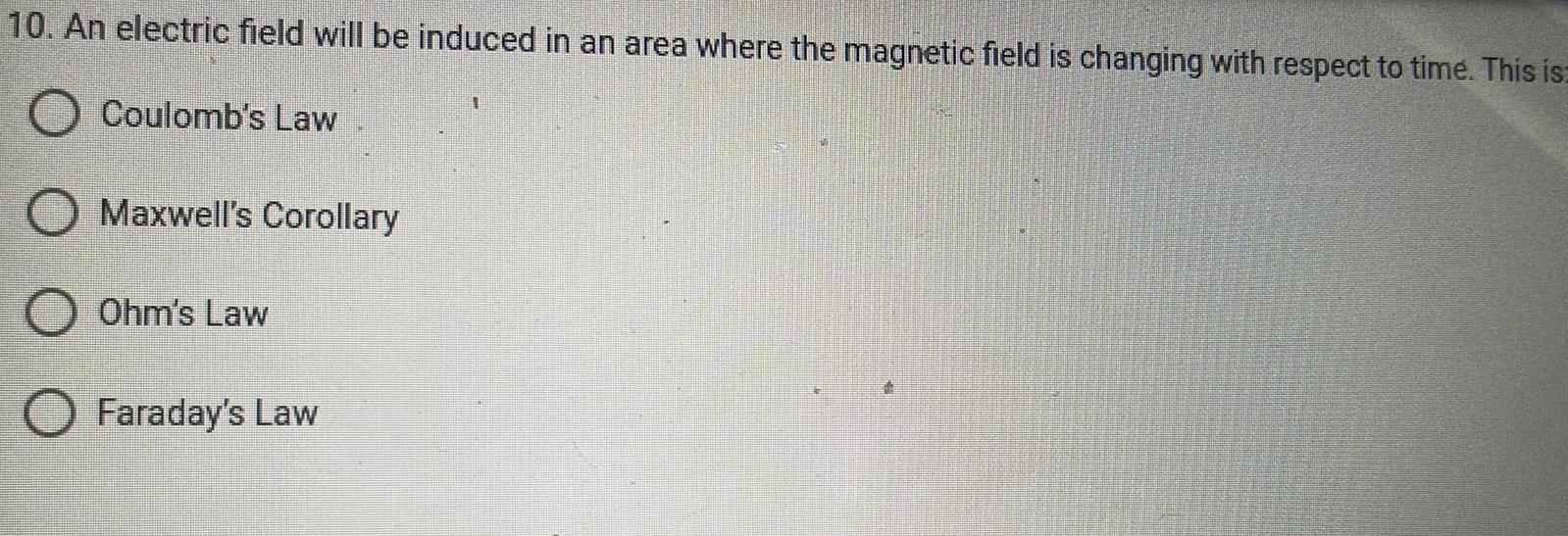Physics
Magnetic Field
An electric field will be induced in an area where the magnetic field is changing with respect to time. This is: Coulomb's Law Maxwell's Corollary Ohm's Law Faraday's Law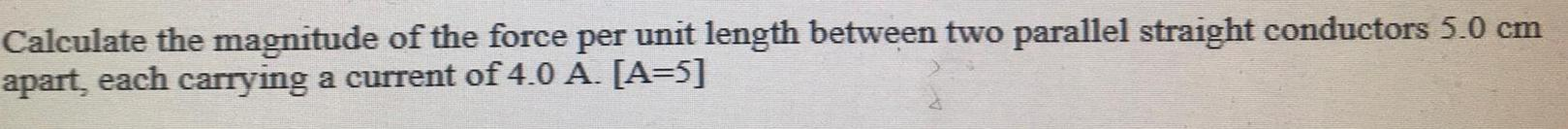Physics
Magnetic Field
Calculate the magnitude of the force per unit length between two parallel straight conductors 5.0 cm apart, each carrying a current of 4.0 A. [A=5]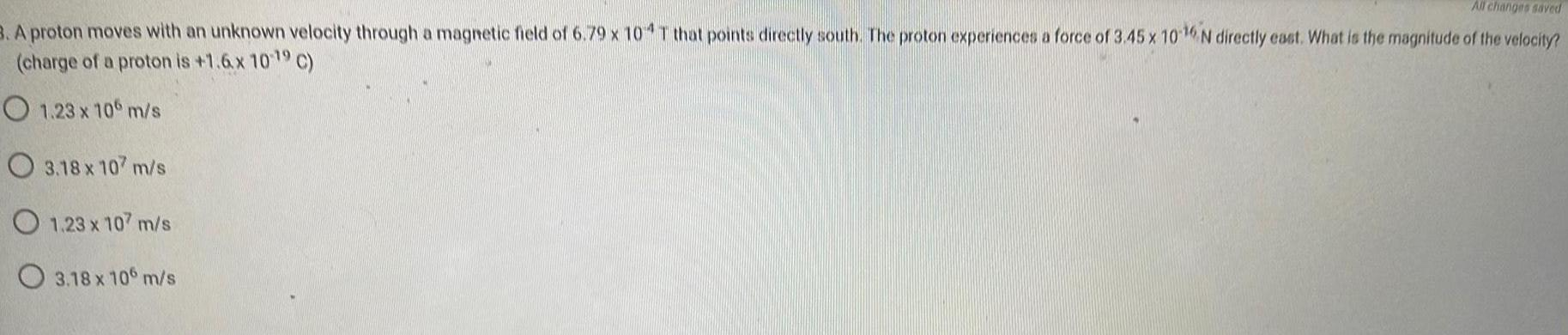Physics
Magnetic Field
A proton moves with an unknown velocity through a magnetic field of 6.79 x 10-4 T that points directly south. The proton experiences a force of 3.45 x 10-16 N directly east. What is the magnitude of the velocity? (charge of a proton is +1.6x 10-19 C) 1.23 x 106 m/s 3.18 x 107 m/s 1.23 x 107 m/s 3.18 x 106 m/s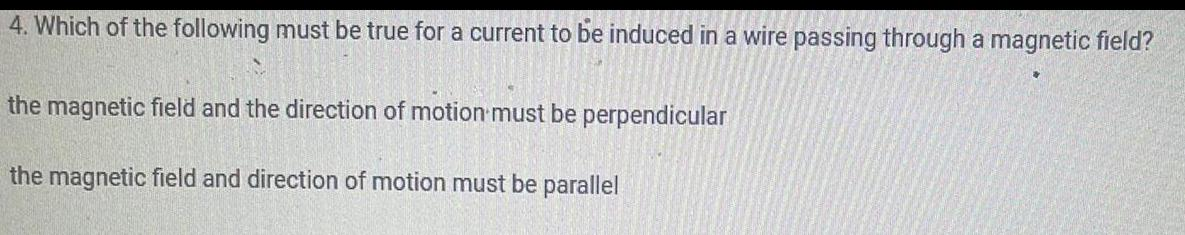Physics
Magnetic Field
4. Which of the following must be true for a current to be induced in a wire passing through a magnetic field? the magnetic field and the direction of motion must be perpendicular the magnetic field and direction of motion must be parallel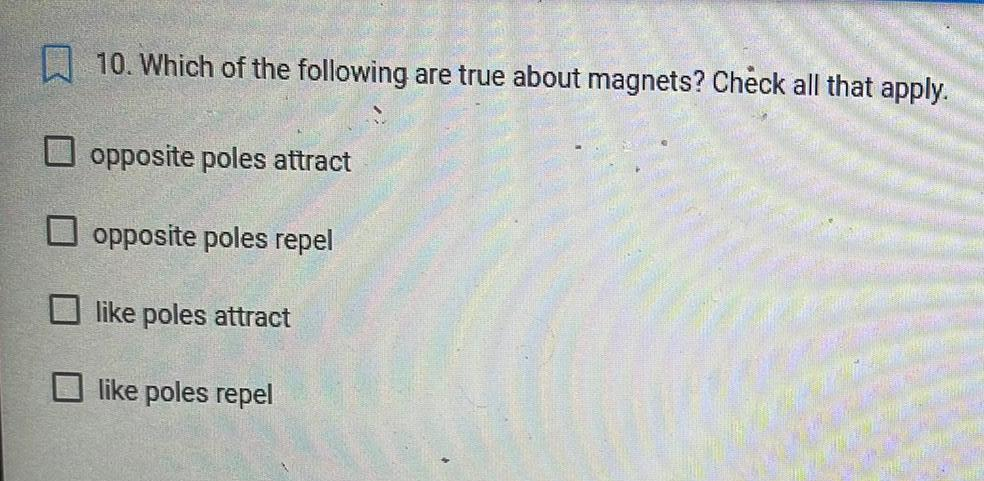Physics
Magnetic Field
10. Which of the following are true about magnets? Check all that apply. opposite poles attract opposite poles repel like poles attract like poles repel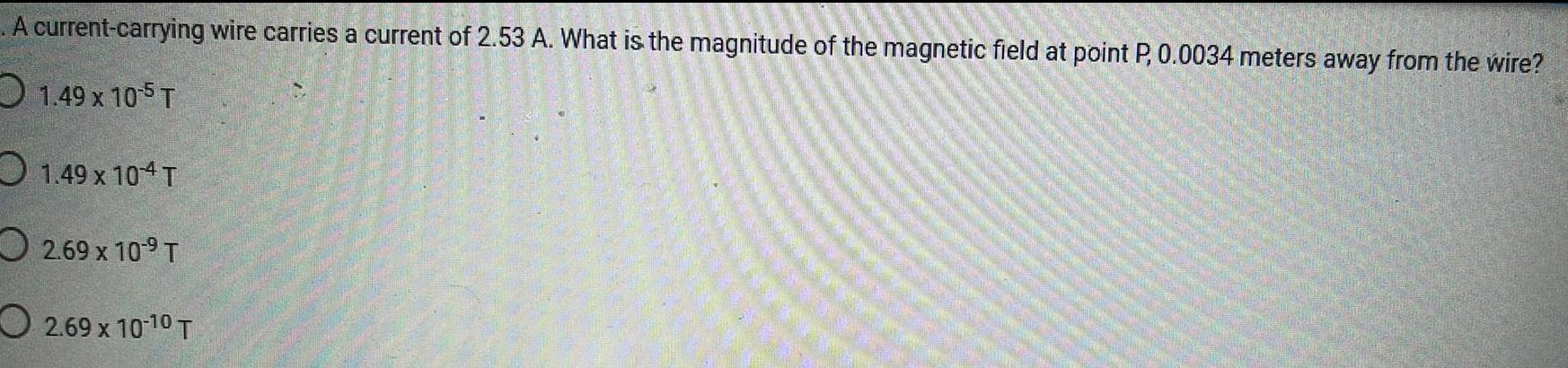Physics
Magnetic Field
A current-carrying wire carries a current of 2.53 A. What is the magnitude of the magnetic field at point P, 0.0034 meters away from the wire? 1.49 x 10-5 T 1.49 x 10-4 T 2.69 x 10-9 T 2.69 x 10-10 T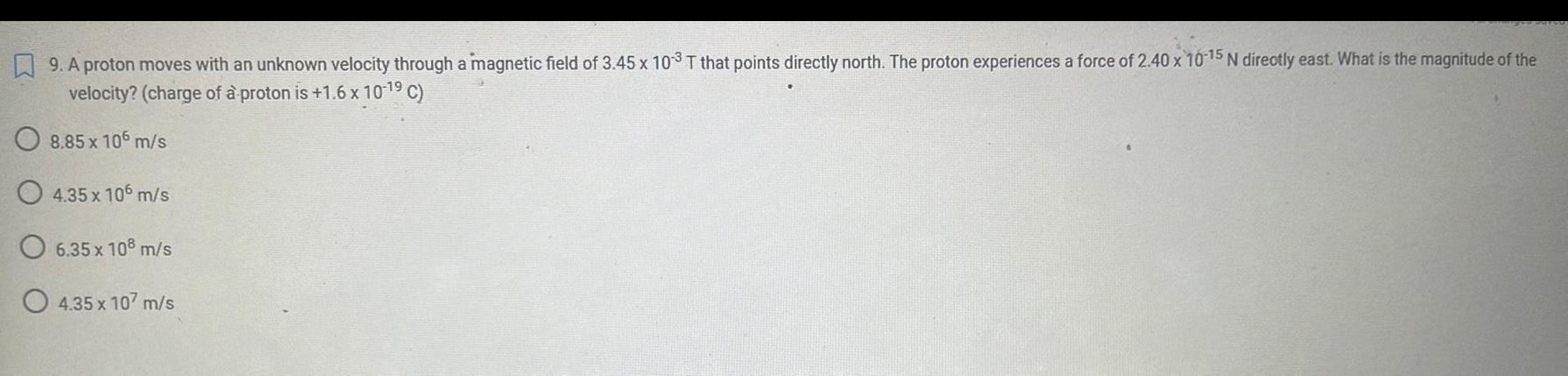Physics
Magnetic Field
9. A proton moves with an unknown velocity through a magnetic field of 3.45 x 103 T that points directly north. The proton experiences a force of 2.40 x 10-15 N directly east. What is the magnitude of the velocity? (charge of a proton is +1.6 x 10-1⁹ C) 8.85 x 106 m/s 4.35 x 106 m/s 6.35 x 108 m/s 4.35 x 107 m/s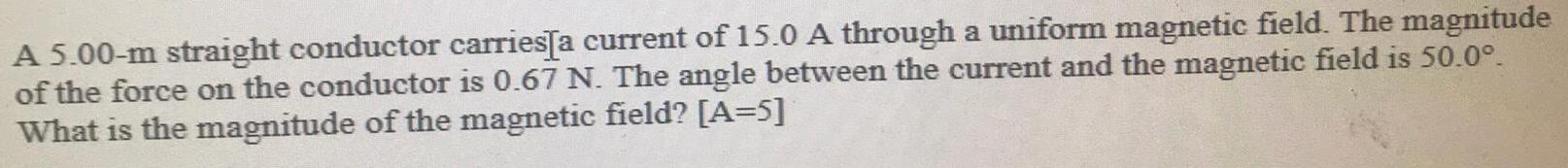Physics
Magnetic Field
A 5.00-m straight conductor carries[a current of 15.0 A through a uniform magnetic field. The magnitudeof the force on the conductor is 0.67 N. The angle between the current and the magnetic field is 50.0°. What is the magnitude of the magnetic field? [A=5]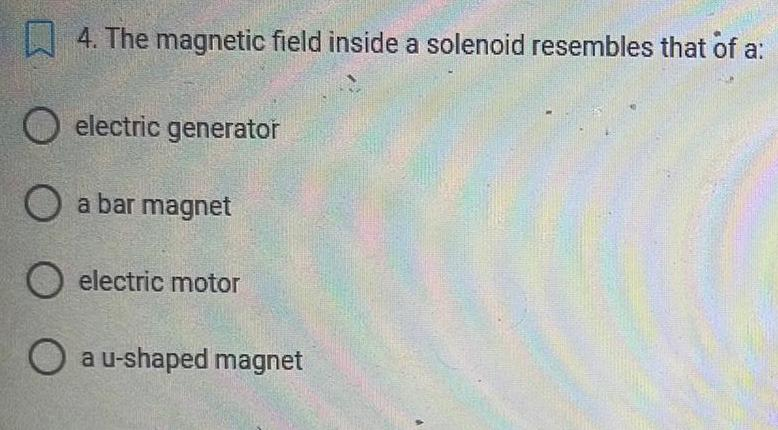Physics
Magnetic Field
4. The magnetic field inside a solenoid resembles that of a: electric generator a bar magnet electric motor a u-shaped magnet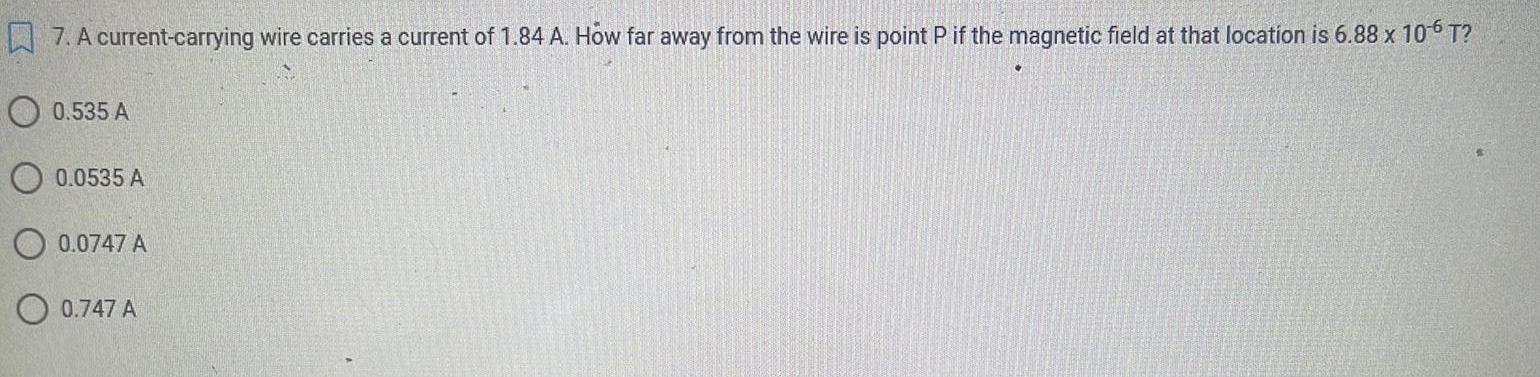Physics
Magnetic Field
7. A current-carrying wire carries a current of 1.84 A. How far away from the wire is point P if the magnetic field at that location is 6.88 x 10-6 T? 0.535 A 0.0535 A 0.0747 A O 0.747 A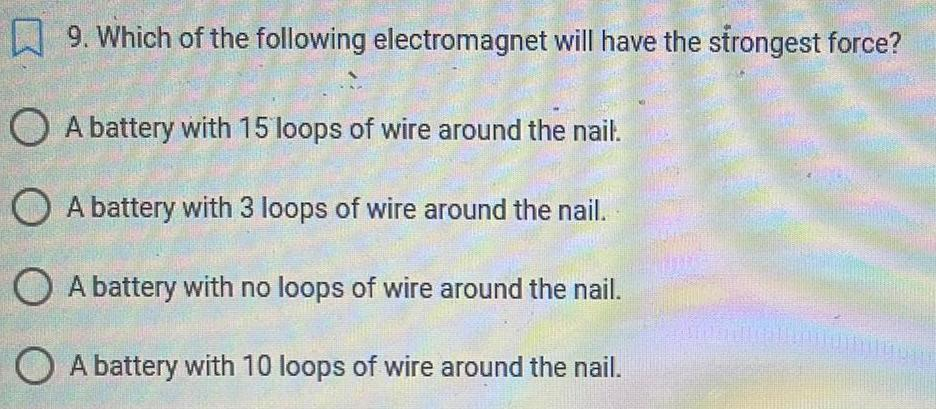Physics
Magnetic Field
9. Which of the following electromagnet will have the strongest force? A battery with 15 loops of wire around the nail. A battery with 3 loops of wire around the nail. A battery with no loops of wire around the nail. A battery with 10 loops of wire around the nail. MALIL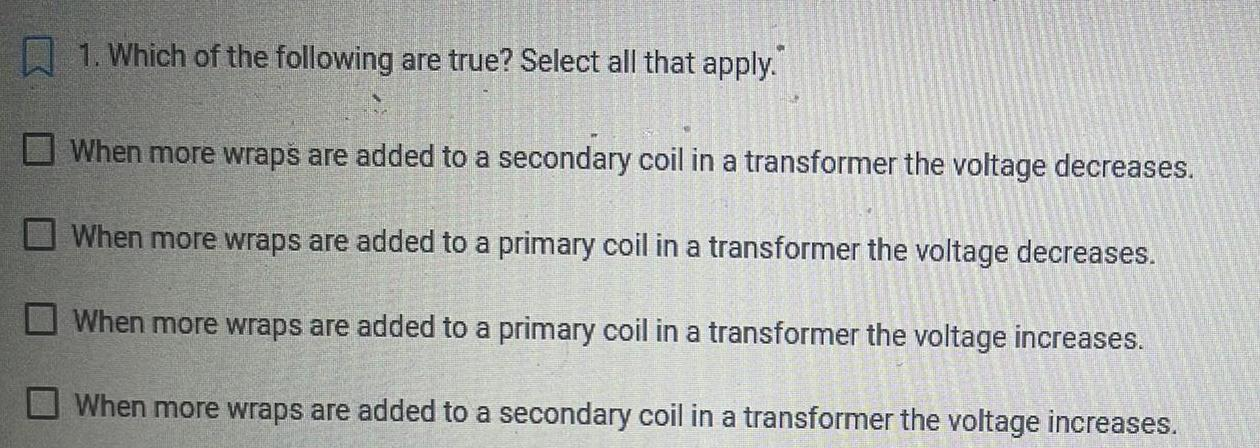Physics
Magnetic Field
1. Which of the following are true? Select all that apply. When more wraps are added to a secondary coil in a transformer the voltage decreases. When more wraps are added to a primary coil in a transformer the voltage decreases. When more wraps are added to a primary coil in a transformer the voltage increases. When more wraps are added to a secondary coil in a transformer the voltage increases.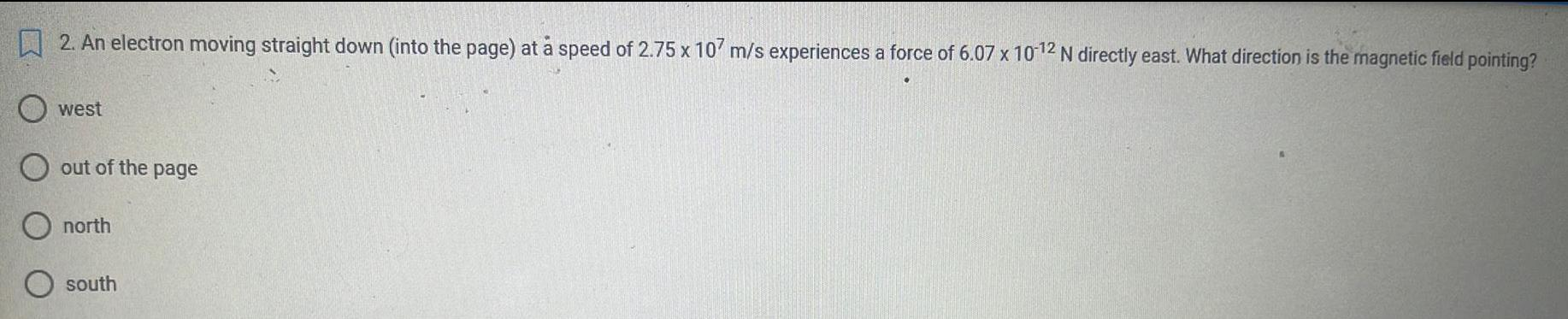Physics
Magnetic Field
2. An electron moving straight down (into the page) at å speed of 2.75 x 107 m/s experiences a force of 6.07 x 10-12 N directly east. What direction is the magnetic field pointing? west out of the page north south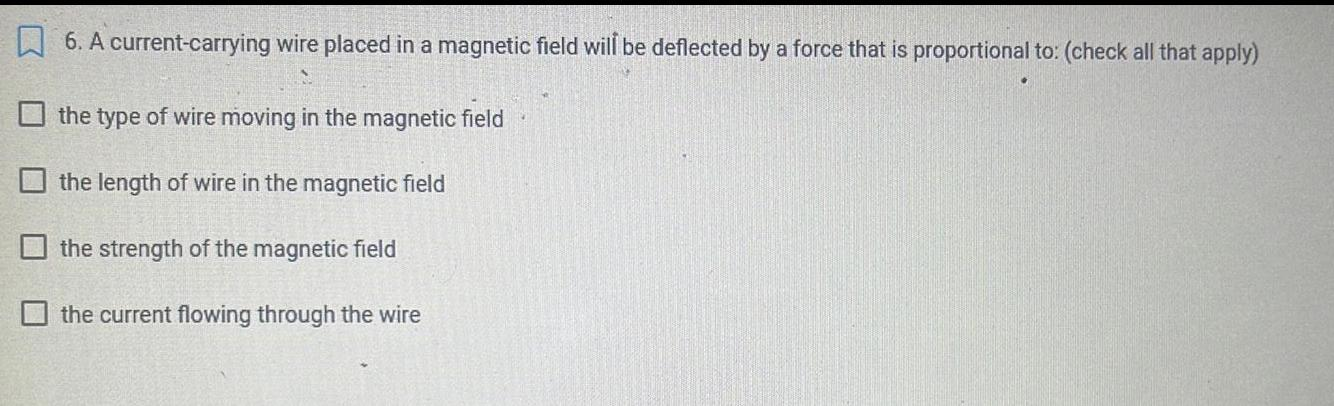Physics
Magnetic Field
6. A current-carrying wire placed in a magnetic field will be deflected by a force that is proportional to: (check all that apply) the type of wire moving in the magnetic field the length of wire in the magnetic field the strength of the magnetic field the current flowing through the wire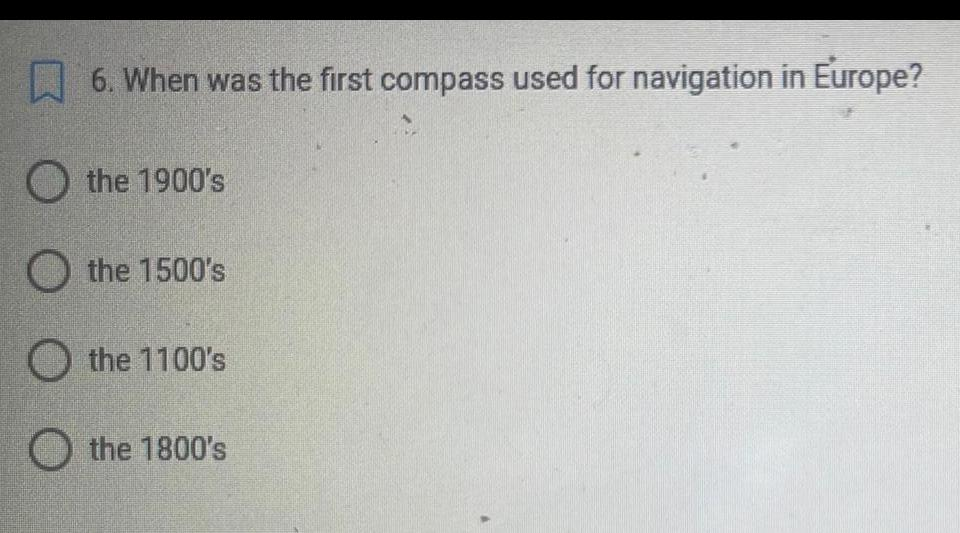Physics
Magnetic Field
6. When was the first compass used for navigation in Europe? the 1900's the 1500's the 1100's the 1800's